#### How to Calculate Z Score in Excel

The z-score, also known as the standard score, is a measurement used in statistics. It is the measurement of the number of standard deviations that a specific number is above or below a mean. The formula to calculating the z-score is:

z = (x – μ) / σ

where:

• z is the z-score,
• x is the value to be standardized,
• μ is the mean of the given set of data,
• σ is the standard deviation of the given set of data.

Suppose you are a student and there are a total of 10 students in your class, including you. After the final exam result is published, you will want to know how well you performed compared to the average student’s mean score. You can find this easily using z-score.

## Calculate z-score

Open Excel and save your file as zscore.xlsx. Type “Name” in A1, “Marks” in B1, “z-score” in C1, “Mean” in F1 and “Standard Deviation” in F2. You should not enter the double quotes when you type in the data. You can format these cells and make them bold. In cells A2 to A11, type ten different names, and in cells B2 to B11, type ten different marks. Now your screen will look like this, though the entries are different.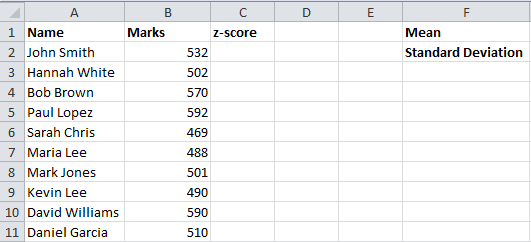Click on cell G1 and go to Formulas (main menu) –> More Functions (in the Function Library group) –> Statistical and select the AVERAGE function.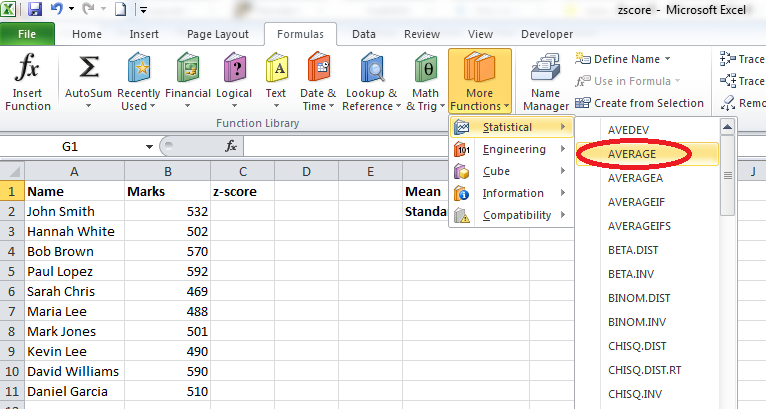You will get a screen like this: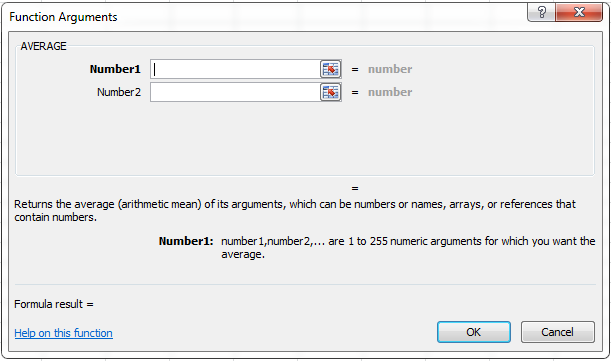In the text box Number1, enter B2:B11 and click OK.

Click on cell G2 and go to Formulas (main menu) –> More Functions (in the Function Library group) –> Statistical and select the STDEVPA function.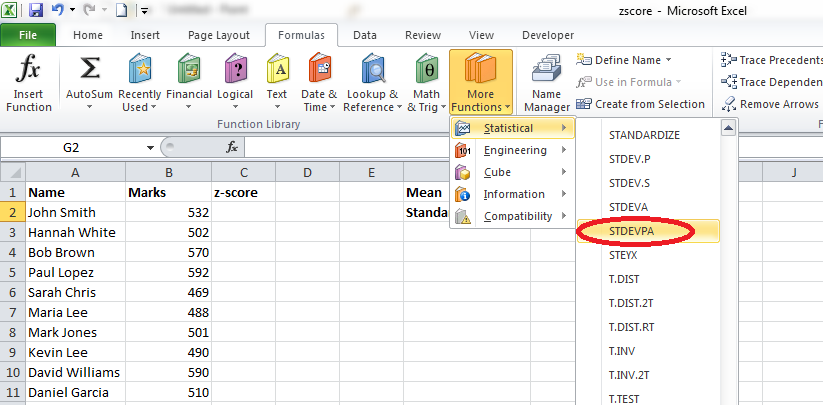You will get a screen like this: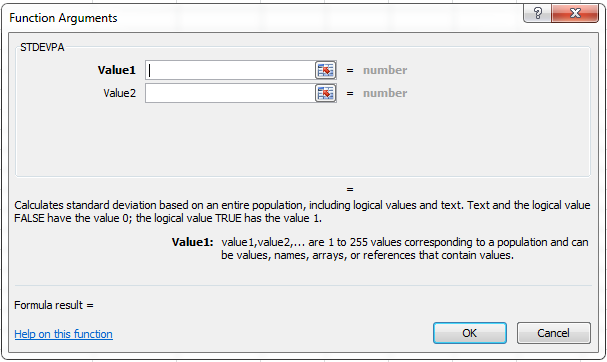In the text box Value1, enter B2:B11 and click OK.

Now your screen will look like this: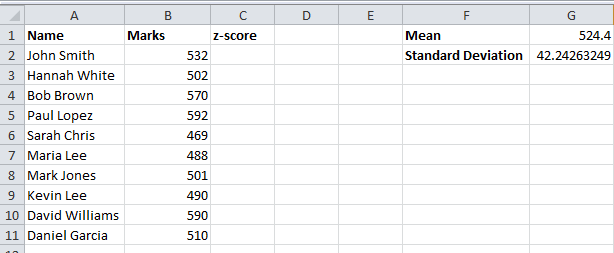Click on cell C2 and go to Formulas (main menu) –> More Functions (in the Function Library group) –> Statistical and select the STANDARDIZE function.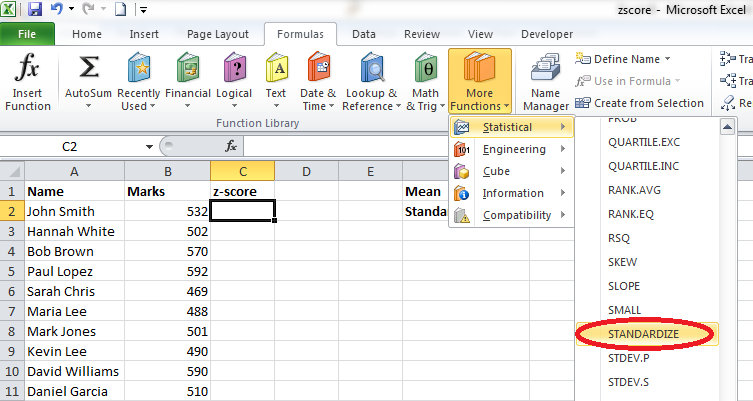You will get a window like this: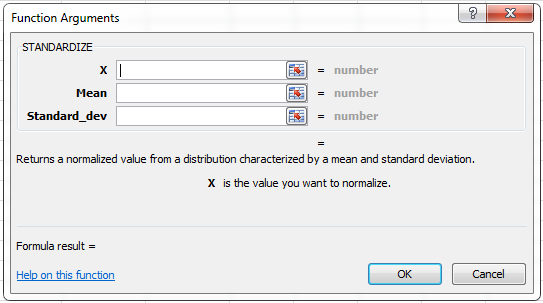Enter “B2” in the X text box, enter “\$G\$1” in the Mean text box, and “\$G\$2” in the Standard_dev text box. Click OK and you will get a value in the cell C2. Copy the formula in cell C2 and paste it into cells C3–C11. Now your screen will look like this. Of course, the values would be different based on your entries.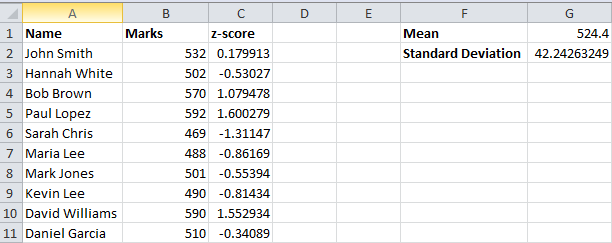Instead of using the STANDARDIZE function, you can directly enter the formula in the address bar like this: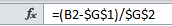In this case, we are using the formula to calculate the z-score directly instead of using the built-in STANDARDIZE function.

If you analyze the data, you will find that the highest z-score value is 1.600279 which is the z-score of Paul Lopez, who scored the highest marks of 592. Sarah Chris has got the lowest z-score and, obviously, the lowest score in the exam.

You can find both positive and negative z-scores. Students who got scores higher than the mean (value in cell G1) get positive z-scores and those who scored less than the mean get negative z-scores. If the z-score of a student is zero, it means that his or her score is the same as the mean value. We can test this easily. Adjust the scores in such a way that one of the students’ scores is the same as the average. Make changes to the scores as shown in the following image: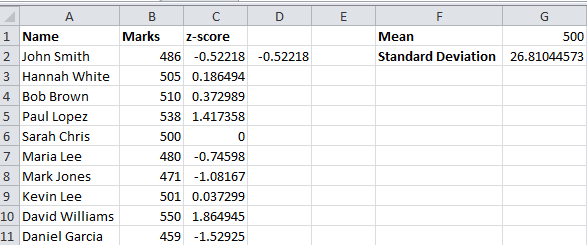Here, you could find that the z-score of Sarah Chris is 0 because her score is the same as the average score. Students who scored more than 500 have a positive z-score and those who scored less than 500 have a negative z-score.

## Using STANDARIZE function

Another method to calculate Z-Score in Excel is by using the STANDARDIZE function. The STANDARDIZE function returns the Z-Score for a given value, based on the mean and standard deviation of the data set.

Here’s how you can use the STANDARDIZE function:

1. Enter the data set into a column in an Excel spreadsheet.
2. Calculate the mean of the data set using the AVERAGE formula: =AVERAGE(range), where range is the range of cells that contain the data set.
3. Calculate the standard deviation of the data set using the STDEV formula: =STDEV(range), where range is the range of cells that contain the data set.
4. In a new cell, enter the STANDARDIZE formula: =STANDARDIZE(x, mean, standard deviation), where x is the value for which you want to calculate the Z-Score, mean is the average of the data set, and standard deviation is the standard deviation of the data set.
5. Press Enter to calculate the Z-Score.

## Using NORMSINV function

Another method to calculate the Z-Score in Excel is by using the NORMSINV function. The NORMSINV function returns the inverse of the standard normal cumulative distribution function for a given value. By combining this function with the standard deviation and mean, you can calculate the Z-Score.

Here’s how you can use the NORMSINV function:

1. Enter the data set into a column in an Excel spreadsheet.
2. Calculate the mean of the data set using the AVERAGE formula: =AVERAGE(range), where range is the range of cells that contain the data set.
3. Calculate the standard deviation of the data set using the STDEV formula: =STDEV(range), where range is the range of cells that contain the data set.
4. In a new cell, enter the following formula: =(x – mean) / standard deviation.
5. In another new cell, enter the NORMSINV formula: =NORMSINV(x), where x is the result from step 4.
6. Press Enter to calculate the Z-Score.

## Using Z.INV function

Another method to calculate the Z-Score in Excel is by using the Z.INV function. The Z.INV function returns the inverse of the standard normal cumulative distribution function for a given probability. By combining this function with the cumulative probability of the Z-Score, you can calculate the Z-Score.

Here’s how you can use the Z.INV function:

1. Enter the cumulative probability of the Z-Score into a cell. This value should be between 0 and 1, exclusive.
2. In a new cell, enter the Z.INV formula: =Z.INV(probability, mean, standard deviation), where probability is the cumulative probability of the Z-Score from step 1, mean is the mean of the standard normal distribution (which is 0), and standard deviation is the standard deviation of the standard normal distribution (which is 1).
3. Press Enter to calculate the Z-Score.

Note: You can copy and paste the formula to calculate the Z-Score for multiple cumulative probabilities.

## Using NORMDIST function

Another method to calculate the Z-Score in Excel is by using the NORMDIST function. The NORMDIST function returns the cumulative probability of the standard normal distribution for a given Z-Score. By combining this function with the cumulative probability of the data set, you can calculate the Z-Score.

Here’s how you can use the NORMDIST function:

1. Enter the cumulative probability of the data set into a cell. This value should be between 0 and 1, inclusive.
2. In a new cell, enter the NORMDIST formula: =NORMDIST(probability, mean, standard deviation, cumulative), where probability is the cumulative probability of the data set from step 1, mean is the mean of the data set, standard deviation is the standard deviation of the data set, and cumulative is a logical value indicating whether the cumulative distribution function is returned (TRUE) or the probability density function is returned (FALSE).
3. In another new cell, enter the formula: =(NORMDIST(probability, mean, standard deviation, TRUE) – 0.5) * SQRT(2) * STDEV.P(range) / AVERAGE(range).
4. Press Enter to calculate the Z-Score.

Note: You can copy and paste the formula to calculate the Z-Score for multiple cumulative probabilities.

## Using LINEST function

Another method to calculate the Z-Score in Excel is by using the LINEST function. The LINEST function returns the statistics for a line that best fits a set of data points. By assuming that the data set follows a normal distribution and using the slope and y-intercept of the best-fit line, you can calculate the Z-Score.

Here’s how you can use the LINEST function:

1. Enter the data set into two columns in an Excel spreadsheet, with one column for the independent variable and another column for the dependent variable.
2. In a new cell, enter the LINEST formula: =LINEST(dependent range, independent range, 1, TRUE), where dependent range is the range of cells that contain the dependent variable, independent range is the range of cells that contain the independent variable, and 1 is a logical value indicating whether to force the y-intercept to be 0 (TRUE) or not (FALSE).
3. Press Enter to calculate the statistics for the best-fit line. The slope of the line is returned in the second element of the resulting array, and the y-intercept is returned in the first element.
4. In another new cell, enter the formula: =(x – mean) / STDEV.P(range), where x is the independent variable for which you want to calculate the Z-Score, mean is the average of the independent variable, and range is the range of cells that contain the independent variable.
5. Press Enter to calculate the Z-Score.

## Using RANK function

Another method to calculate the Z-Score in Excel is by using the RANK function in combination with the AVERAGE and STDEV.P functions. The RANK function returns the rank of a given value in a set of values, while the AVERAGE and STDEV.P functions return the average and standard deviation of a set of values, respectively. By using these functions, you can calculate the Z-Score as the difference between a value and the average of the set, divided by the standard deviation of the set.

Here’s how you can use these functions:

1. Enter the data set into a column in an Excel spreadsheet.
2. In a new cell, enter the formula: =(x – AVERAGE(range)) / STDEV.P(range), where x is the value for which you want to calculate the Z-Score, and range is the range of cells that contain the data set.
3. Press Enter to calculate the Z-Score.

## Using Z.TEST function

The Z.TEST function is another method to calculate the Z-Score in Excel. The Z.TEST function returns the probability of a standard normal distribution for a given Z-Score. By assuming that the data set follows a normal distribution, you can use the Z.TEST function to calculate the Z-Score and determine how likely it is that a particular value comes from the set.

Here’s how you can use the Z.TEST function:

1. Enter the data set into a column in an Excel spreadsheet.
2. In a new cell, enter the Z.TEST formula: =Z.TEST(range, x), where range is the range of cells that contain the data set and x is the value for which you want to calculate the Z-Score.
3. Press Enter to calculate the probability of the standard normal distribution for the Z-Score. The resulting value is a decimal between 0 and 1 that represents the probability that the Z-Score comes from the set.

Note: You can use the Z.TEST function to calculate the Z-Score and determine the probability of the standard normal distribution for multiple values in the data set. To find the Z-Score itself, you would need to use one of the other methods described in above examples.

In conclusion, there are several methods for calculating the Z-Score in Excel, including the =STANDARDIZE function, the combination of the =RANK, =AVERAGE, and =STDEV.P functions, and the =Z.TEST function. Each method offers a different approach to finding the Z-Score and can be used depending on the specific requirements of your data analysis. The Z-Score is a valuable tool for understanding how values in a data set relate to the mean and standard deviation of the set, and can be used in a variety of applications, including statistical analysis, quality control, and outlier detection.

## Key Takeaways

• The z-score is a measurement used in statistics to determine how far a specific number is from the mean of a set of data.
• The z-score is calculated by subtracting the mean from the number and then dividing by the standard deviation.
• The z-score can be used to compare different sets of data that have different means and standard deviations.
• The z-score can also be used to determine the probability of a particular number occurring in a set of data.

## FAQ

• Q: What is the difference between the z-score and the standard deviation?
• A: The z-score is a standardized measure of the standard deviation, while the standard deviation is a measure of how spread out the data is.
• Q: How can I calculate the z-score in Excel?
• A: There are several ways to calculate the z-score in Excel. You can use the STANDARDIZE function, the NORMSINV function, the Z.INV function, the NORMDIST function, the LINEST function, or the RANK function.
• Q: What are the applications of the z-score?
• A: The z-score can be used in a variety of applications, including: * Comparing different sets of data * Determining the probability of a particular number occurring in a set of data * Identifying outliers in a set of data * Determining the significance of a difference between two means